Module 15 - Income Determination Flashcards Preview

Economics > Module 15 - Income Determination > Flashcards

Flashcards in Module 15 - Income Determination Deck (40):
1

2

Using the compound interest formula if a principal sum P0 were lent at interest rate R for two years the principal sum received at the end of two years P2 would be P2 = P0(1 + R)(1 + R) or P2 = P0(1 + R)2 Which of the following is the principal to be received at the end of n years? A. Pn = P0(1 + R) × n B. Pn = P0(1 + R)n C. Pn = n(1 + R)n D. Pn = n(1 + R)3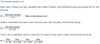4

Which of the following is correct? The diagram above indicates that the MEI A. depends upon the interest rate R B. becomes negative if R decreases from R2 to R1 C. declines as the value of investment undertaken increases D. and R are in equilibrium only at a zero rate5

Which of the following is correct?If R were zero the equilibrium level of investment would beA. OI0 or OI1 or OI2 B. OI0 only C. Zero D. Infinite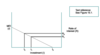6

If r were zero this would indicate that I. the rate of interest was zero II. all investments with a positive r had been undertaken III. the MEI function was a horizontal line Which of the following is correct? A. I and III only B. II only C. I, II and III D. Not I, not II, not III7

8

Y ≡ C + I + G C = .75Yd Yd = Y − TAssuming sufficient unemployed resources to allow all multiplier rounds to be completed.Which of the following is the impact on Y of an increase in G of 10?A. 10 B. 20 C. 30 D. 409

10

11

The slope of the MEI curve assumes that as the volume of investment increases the marginal efficiency of investment will decline. Over time, however, there is no empirical evidence to suggest that the rate of return over cost has declined. These apparently conflicting views can be resolved because I. the slope of the MEI curve assumes technical knowledge is fixed in the short-run II. the rate of interest has declined over time III. advances in technology shift the MEI schedule to the right over time Which of the following is correct? A.I only B. I and III only C. I, II and III D. Not I, not II, not III12

Which of the following factors will shift the MEI schedule to the left?A. Anticipation of a world wide boom B. Breakdown of world trade agreement and increases in tariffs and quotas C. Decrease in raw materials prices D. Research indicating higher than expected future returns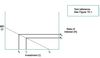13

Which of the following factors will shift the MEI schedule to the right? Increase in A. the degree of uncertainty regarding future returns B. the rate of interest C. the expected returns on investment D. costs of doing business due to adherence to international standards14

15

The accelerator principle does not yield a comprehensive explanation of investment expenditure but it does deal with investment associated withI. inventions and technological change II. depreciation of the capital stock III. changes in the rate of interestWhich of the following is correct? A. I and II only B. II and III only C. I, II and III D. Not I, not II, not III16

Which of the following values of the marginal propensity to consume and the accelerator combined will result in the smallest fluctuation in income, output and employment?17

Y = C + I + GC = 0.75ydyd = y(1-t)t = 0.2Which of the following is the impact on Y of an increase in G of 10?A. 10 B. 25 C. 50 D. 100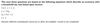18

Y = C + I + GC = 0.75ydyd = y(1-t)t = 0.2Which of the following is the impact on Y of a decrease in I of 20?A. 0 B. −20 C. −50 D. −10019

Y = C + I + GC = 0.75ydyd = y(1-t)t = 0.2Which of the following is the impact on Y of an increase in G of 11 accompanied by an increase in the tax rate from 20% to 40%?A. 11 B. 20 C. 22 D. 3620

21

Which of the following is correct? A. Y = C + I + G B. Y = C + I + G − T C. Y = C + I + G + T D. Y = (C + I + G + T) / 0.822

Which of the following is the equilibrium level of national income? A. 2400. B. 2750. C. 2775. D. 2875.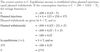23

Which of the following is true of the given simple economy? A. The chief cause of unemployment is insufficient spending. B. The equilibrium level of national income coincides with the full-employment level. C. Inflation and employment are negatively related. D. Tax cuts are more powerful than government spending in increasing employ- ment.24

25

26

Which of the following could have caused the shift in the investment level from I1 to I2? A. A decrease in the rate of interest. B. An increase in the business tax rate. C. An increase in the rate of interest. D. Labour-saving innovations.27

In period 1 investment was 0i1 and it remained at that level in period 2, despite the shift in the investment function. Which of the following could account for this? A. The demand for replacement investment increased. B. The rate of interest increased from 15 per cent to 20 per cent. C. Firms delayed investment by the amount of i1i2. D. Imports of investment goods increased.28

The rate of interest was 15 per cent in period 1 and 10 per cent in period 2. Which of the following represents the change in investment between the two periods? A. From 0i1 to 0i3. B. From 0i2 to 0i3. C. From 0i3 to 0i1. D. From 0i2 to 0i1.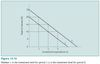29

30

31

32

33

34

35

36

37

38

39

40